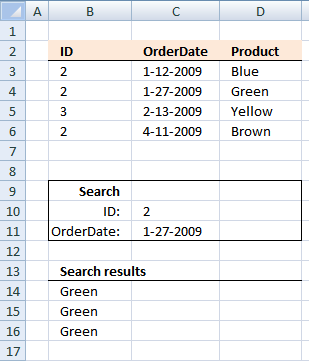Author: Oscar Cronquist Article last updated on October 28, 2018Formula in B14:

=INDEX(D3:D6, SUMPRODUCT(--(C10=B3:B6), --(C11=C3:C6), ROW(D3:D6)-MIN(ROW(D3:D6))+1))

Alternative array formula #1 in B15:

=INDEX(D3:D6, MATCH(C10&"-"&C11, B3:B6&"-"&C3:C6, 0))

Alternative array formula #2 in B16:

=INDEX(D3:D6, MIN(IF((C10=B3:B6)*(C11=C3:C6), ROW(D3:D6)-MIN(ROW(D3:D6))+1)))

Alternative array formula #3 in B17:

=INDEX(D3:D6, MATCH(1,COUNTIFS(C10,B3:B6,C11,C3:C6),0))

Alternative array formula #4 in B18:

=INDEX(D3:D6, MATCH(1,(C10=B3:B6)*(C11=C3:C6),0))

How to enter an array formula

1. Select a cell
2. Type array formula in formula bar
3. Press and hold CTRL + SHIFT keys simultaneously
4. Press ENTER

If you did the above steps correctly the formula begins and ends with a curly bracket, like this {=array_formula}

### Explaining array formula in cell B18

Step 1 - Compare value in C10 with values B3:B6 and C11 with C3:C6 and then multiply arrays

(C10=B3:B6)*(C11=C3:C6)

becomes

{TRUE;TRUE;FALSE;TRUE}*{FALSE;TRUE;FALSE;FALSE}

and returns

{0;1;0;0}

Step 2 - Match 1 against array

MATCH(1,(C10=B3:B6)*(C11=C3:C6),0)

becomes

MATCH(1,{0;1;0;0},0)

and returns 2.

Step 3 - Return corresponding product

INDEX(D3:D6, MATCH(1,(C10=B3:B6)*(C11=C3:C6),0))

becomes

INDEX({"Blue";"Green";"Yellow";"Brown"}, 2)

and returns Green in cell B18.

### Get excel file for this tutorial.

Lookup two index columns.xls
(Excel 97-2003 Workbook *.xls)

MATCH(lookup_value, lookup_array, [match_type])
Returns the relative position of an item in an array that matches a specified value

INDEX(array,row_num,[column_num])
Returns a value or reference of the cell at the intersection of a particular row and column, in a given range

MIN(number1,[number2])
Returns the smallest number in a set of values. Ignores logical values and text

ROW(reference) returns the rownumber of a reference

IF(logical_test, [value_if_true], [value_if_false])
Checks whether a condition is met, and returns one value if TRUE, and another value if FALSE

SUMPRODUCT(array1, array2, )
Returns the sum of the products of the corresponding ranges or arrays

COUNTIFS(criteria_range1,criteria1, criteria_range2, criteria2...)
Counts the number of cells specified by a given set of conditions or criteria